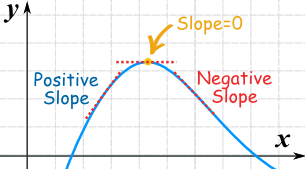# How do I find the absolute maximum and minimum of a function?

Mar 1, 2017

You find where the derivative is zero

#### Explanation:

STEP ONE
Find two things:

$$ 1. The endpoints
2. The places where the slope is zero


The end points could be the maximum or minimum because we don't know where the function starts or finishes

If the slope is zero, you know that there is a relative maximum or minimum. We need to check if it is the absolute maximum or minimum or not.[From mathisfun.com, because math is fun]

$| \textcolor{\lim e}{\text{You can find where the slope is zero...}}$
$| \textcolor{\lim e}{\text{by setting the derivatives of the function to zero}}$

The derivative is a function of the slope. Thus, if you can see the derivative to zero, you can solve for all the relative max or min points.

STEP TWO

Plug each possible max or min point into the original function (not the derivative because we do not care about the slope anymore), and see which one is the largest and which one is the smallest.

Some function have multiple absolute maximums and minimums, especially trig functions.